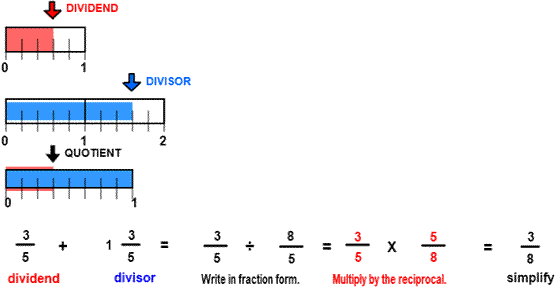# Divide and simplify fractions

## Divide Fractions and Simplify with Number Line Models

DIVIDE AND SIMPLIFY
CORRECT:
ATTEMPTS:
SCORE:
PERCENT
WHOLE
NUMERATOR
DENOMINATOR

# DIVIDE AND SIMPLIFY FRACTIONS INSTRUCTIONS

Follow the directions in the dialog box after pressing the <START> button. The <EXPLAIN> button may then be pressed after you enter the divisor to see how to do the example.

The following image was made by Divide with Lines Designer:Divide Fractions and Simplify is similar to the previous program DIVIDE FRACTIONS except that the divisor may be larger than the dividend and the quotient may be a mixed number.

You can see from the image that 38 of the divisor will fit into the dividend. To see how this happens, think of 1 35 as 85. The numerator of the divisor 8 will fit 38 times into the numerator of the dividend 3.

Another important idea: When the divisor is smaller than the dividend, the quotient is larger than 1. When the divisor is equal to the dividend, the quotient is equal to 1. When divisor is larger than the dividend, the quotient is smaller than 1.

See the program MIXED NUMBERS for information on writing fractions in mixed form.

See the program RENAME IN LOWEST TERMS for information on writing fractions in lowest terms.

To calculate the quotient first write the dividend and the divisor in fraction form as shown in the example above. Then multiply 35 by the reciprocal of the divisor. The reciprocal of the divisor is found by  replacing the numerator with the denominator and replacing the denominator with the numerator. In short, dividing by 85 can be done by multiplying by 58.

See MULTIPLY FRACTIONS  for instructions on how to multiply fractions.

See MULTIPLICATIVE INVERSE for more information on how to find the inverse or reciprocal.

The quotient must be written in simplest form as a mixed number and in lowest terms, if necessary.

For more instruction on dividing fractions go to How To Divide Fractions

After you enter the quotient you may press the <REPORT> button. The report will ask for your name but you may submit a code for your name. This report will give the same results as on the dialog box. The report may be printed or e-mailed.